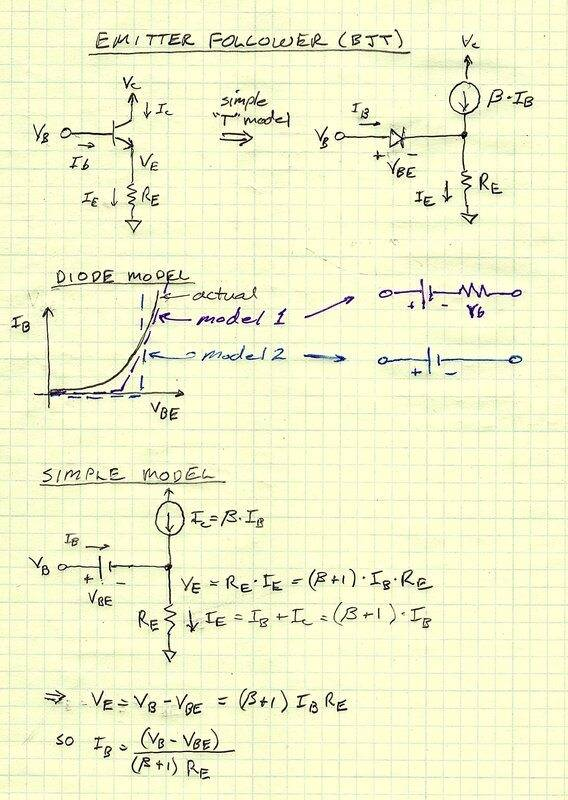# BJT common collector

• Helena Wells
In summary, the transistor will melt from too much current before it saturates when connected to a 5V supply.f

#### Helena Wells

TL;DR Summary
This thread is about the negative feedback of a emitter follower circuit
Assume we have a common collector circuit with a emitter resistance of 1k Ohms.Vc = 10V Ve is connected to ground.

Now we connect the base of the transistor to 5V . The base current will be 5-VBE/1000 Amp.Let's say a voltage drop of 0.7 in the BE junction .We will get Ib = 4.3mA.

Ic = bxIb = 100xIb = 430 mA. Now the voltage drop on the diode becomes 0.43x1000 = 430V . How is that even possible?The BJT must saturate and emitter resistor must have a voltage of 9.8V . But by not putting a base resistor , the BJT saturates?

Even if we put a base resistors the voltage at the emitter current seems to be increased. What is happening?

Last edited:
Summary:: This thread is about the negative feedback of a emitter follower circuit

Now the voltage drop on the diode becomes 0.43x1000 = 430V

No. Diodes are very non-linear. As their current increases the voltage drop mostly stays the same, the increase is small. Look up the I-V curves for a PN diode and you will see this clearly. Usually, we would just assume that it doesn't change at all once it has current flowing through it.

Also keep in mind that we would almost always separate the DC (biasing) model characteristics from the AC (small-signal) characteristics, because we care about different things. In particular the local slope dv/di or ac resistance of the forward bias junction is different than the global slope (V2-V1)/(I2-I1).

It sounds like your circuit has no series resistors anywhere, just a transistor with a Vce fixed supply voltage. In this DC case the collector current is only limited by the amount of base current and the gain. The device will melt from too much current before it saturates.

The emitter follower can be very simple to understand, yet at the same time, quite difficult. In your case, keep in mind you have the base connected to a voltage source. So, you are not really defining the base current until you specify an emitter resistor. That part right there is part of why the emitter follower has negative feedback. In your hypothetical case, the emitter voltage attempts to go very high because of the way the math works out. But, to get that high, the base emitter junction voltage shrinks down to the point that the emitter current drops. Eventually, it settles into a happy medium. That's my 2¢>

If we have 4.3mA collector current, then the base current must be 4.3/100. The voltage this develops across B-E is just a characteristic of the diode and is only roughly 0.6 volt. The 4.3mA will provide 4.3 volts across the emitter load resistor and will require about (5 - 0.6) = 4.3 volts on the base.

It's not clear when you say "emitter resistor" if that is part of your BE junction model or a separate resistor between the emitter and ground. A schematic is always helpful for electronics questions!

I really don't understand some of the answers.Isn't Ib = VCB - 0.7/Re+Rb? And Ic = Ib *β?

Base current in real world practical transistor circuits is seldom nailed down to a specific value. The use of negative feedback with an emitter resistor is what allows this.

Ok thank you!

The physics of transistors can be pretty complex. But, in circuit analysis, we usually avoid most of the complexity by substituting simple circuit models that describe the behavior we care most about. Start with the simplest models, then if there is some slight inaccuracy, you would repeat the analysis with a more complex model.

Here you see my simple analysis of the emitter follower. In this example, first I calculated Ie, then Ve, then Ib. BTW, it's called emitter follower because the emitter voltage is virtually the same as the base voltage minus a fixed drop.

edit: you have to first assume that the circuit is operating in the linear region, i.e. not saturated or reverse biased. After you do the analysis, you can check this assumption. For example, if Vc = 12V but you calculate Ve = 25V, you know that the device won't behave as modeled, the original assumption was wrong and you repeat the analysis a different way.In your example (Vb=5V, β=100, Re=1000, Vc=10V), once you calculate Ve=430V, you can see that the model was wrong. Ve can't rise above (Vb-Vbe) without turning off the diode in the base emitter junction. Also Ve can't rise above Vc (actually Vc - Vce(sat), or about 9.6V) for this model. Then you would repeat the analysis with Ve = Vb - Vbe or about 4.3V, then Ie = 4.3mA and Ib=Ie/(β+1)=4.26uA 42.6uA.

Oops! Fixed typo.

Last edited:
•alan123hk